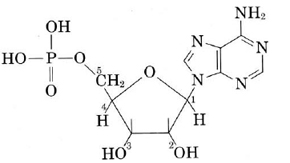# 20.18: Nucleic Acid Structure

$$\newcommand{\vecs}{\overset { \rightharpoonup} {\mathbf{#1}} }$$ $$\newcommand{\vecd}{\overset{-\!-\!\rightharpoonup}{\vphantom{a}\smash {#1}}}$$$$\newcommand{\id}{\mathrm{id}}$$ $$\newcommand{\Span}{\mathrm{span}}$$ $$\newcommand{\kernel}{\mathrm{null}\,}$$ $$\newcommand{\range}{\mathrm{range}\,}$$ $$\newcommand{\RealPart}{\mathrm{Re}}$$ $$\newcommand{\ImaginaryPart}{\mathrm{Im}}$$ $$\newcommand{\Argument}{\mathrm{Arg}}$$ $$\newcommand{\norm}{\| #1 \|}$$ $$\newcommand{\inner}{\langle #1, #2 \rangle}$$ $$\newcommand{\Span}{\mathrm{span}}$$ $$\newcommand{\id}{\mathrm{id}}$$ $$\newcommand{\Span}{\mathrm{span}}$$ $$\newcommand{\kernel}{\mathrm{null}\,}$$ $$\newcommand{\range}{\mathrm{range}\,}$$ $$\newcommand{\RealPart}{\mathrm{Re}}$$ $$\newcommand{\ImaginaryPart}{\mathrm{Im}}$$ $$\newcommand{\Argument}{\mathrm{Arg}}$$ $$\newcommand{\norm}{\| #1 \|}$$ $$\newcommand{\inner}{\langle #1, #2 \rangle}$$ $$\newcommand{\Span}{\mathrm{span}}$$

Nucleic acids were first isolated from cell nuclei (hence the name) in 1870. Since then they have been found in other portions of cells as well, especially the ribosomes, which are the sites of protein synthesis. Most nucleic acids are extremely long-chain polymers—some forms of DNA have molecular weights greater than 109. Nucleic acids are made up from three distinct structural units. These are:

1 A five-carbon sugar. Only two sugars are involved. These are ribose (used in RNA) and deoxyribose (used in DNA). Their structures are shown in Figure $$\PageIndex{1}$$. Note that deoxyribose, as its name implies, has one oxygen less than ribose in the 2 position.Figure $$\PageIndex{1}$$ The structure of the monosaccharides (a) ribose and (b) deoxyribose found in nucleic acids.

2 A nitrogenous base. There are five of these bases. All are shown in Figure $$\PageIndex{2}$$. Three of them, adenine, guanine, and cytosine, are common to both DNA and RNA. Thymine occurs only in DNA, and uracil only in RNA.Figure $$\PageIndex{2}$$ Structures of the principal nitrogenous bases obtained by hydrolysis of nucleic acids. The hydrogen lost when the base is condensed with a sugar is shown in color. (Thymine occurs only in DNA, while the very similar uracil occurs in its place in RNA. Adenine, guanine, and cytosine occur in both DNA and RNA.)

3 Phosphoric acid. H3PO4 provides the unit that holds the various segments of the nucleic acid chain to each other.

The combination of a sugar and a nitrogenous base is called a nucleoside. A typical example of a nucleoside is adenosine, derived by the condensation of ribose and adenine:Nucleosides in turn can be condensed with phosphoric acid by means of the —OH group at carbon atom 5. The result is a structure called a nucleotide. Thus when adenosine condenses with phosphoric acid, the nucleotide formed is adenosine-5-monophosphoric acid, usually indicated by the acronym AMP:Figure $$\PageIndex{3}$$. Note that directionality is introduced along the chain because a sugar connected to one phosphate through carbon atom 5 always joins to the next phosphate via carbon atom 3.Figure $$\PageIndex{3}$$ (a) A portion of a ribonucleic acid chain. (In DNA the oxygens marked in color would not be present and thymine would replace uracil at the two positions where the latter appears along the chain.) (b) Locations of sugar, phosphate, and nitrogenous bases. (c) Representation of each base as a letter of the alphabet.